# Algebra II : Graph Square Root, Cube Root, and Piecewise Functions

## Example Questions

← Previous 1

### Example Question #1 : Graph Square Root, Cube Root, And Piecewise Functions

State the domain of the function: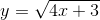Possible Answers: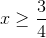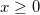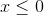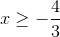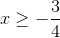Correct answer:Explanation:

Since the expression under the radical cannot be negative,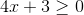.

Solve for x:This is the domain, or possiblevalues, for the function.

### Example Question #2 : Graph Square Root, Cube Root, And Piecewise Functions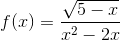Which of the following choices correctly describes the domain of the graph of the function?

Possible Answers: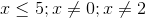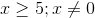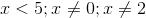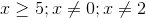All real numbers

Correct answer:Explanation:

Because the term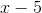inside the radical in the numerator has to be greater than or equal to zero, there is a restriction on our domain; to describe this restriction we solve the inequality for: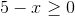Addto both sides, and the resulting inequality is: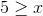or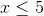Next, we consider the denominator, a quadratic; we know that we cannot divide by zero, so we must find x-values that make the denominator equal zero, and exclude them from our domain: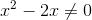We factor anout of bothand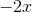: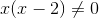Finding the zeroes of our expression leaves us with: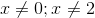Therefore, there are 3 restrictions on the domain of the function:### Example Question #3 : Graph Square Root, Cube Root, And Piecewise Functions

Which of the following is the graph of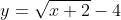?

Possible Answers: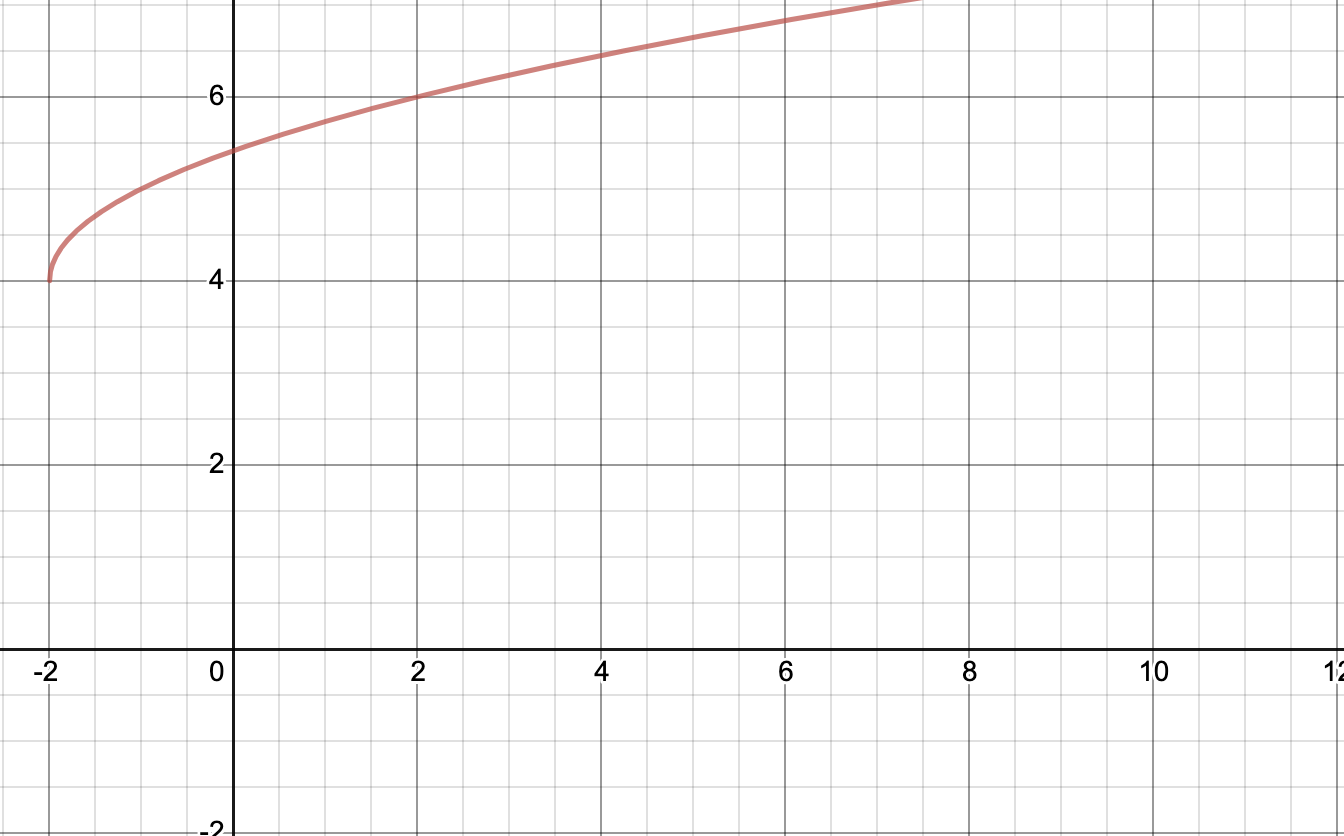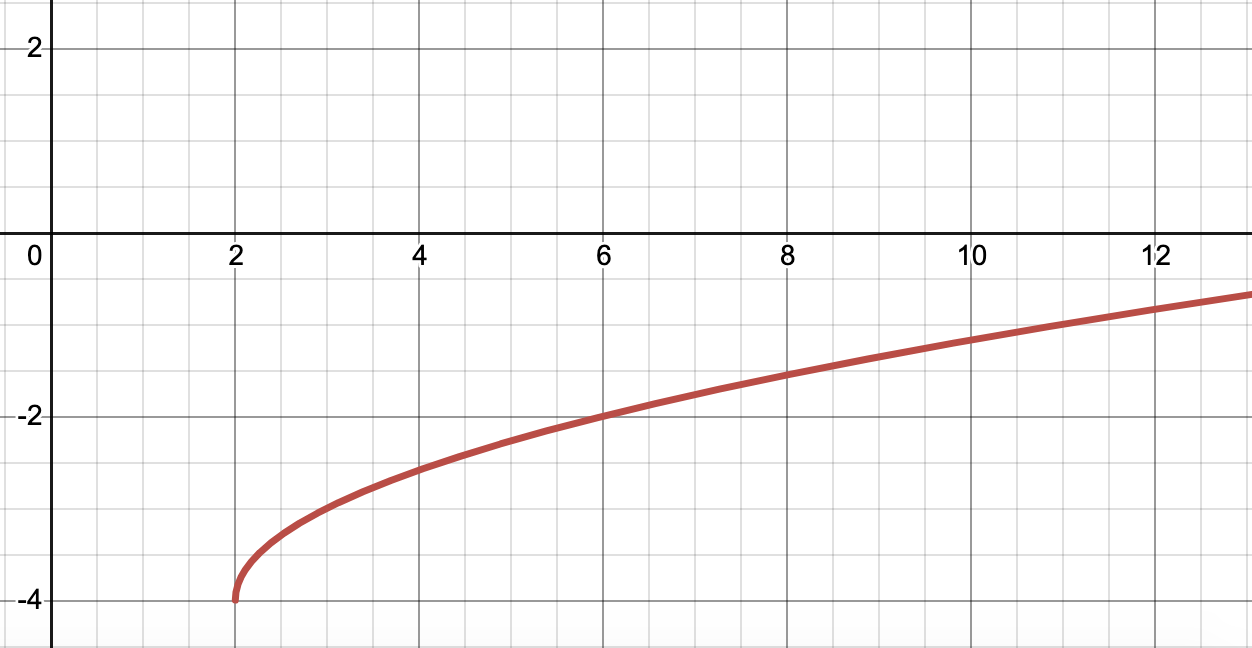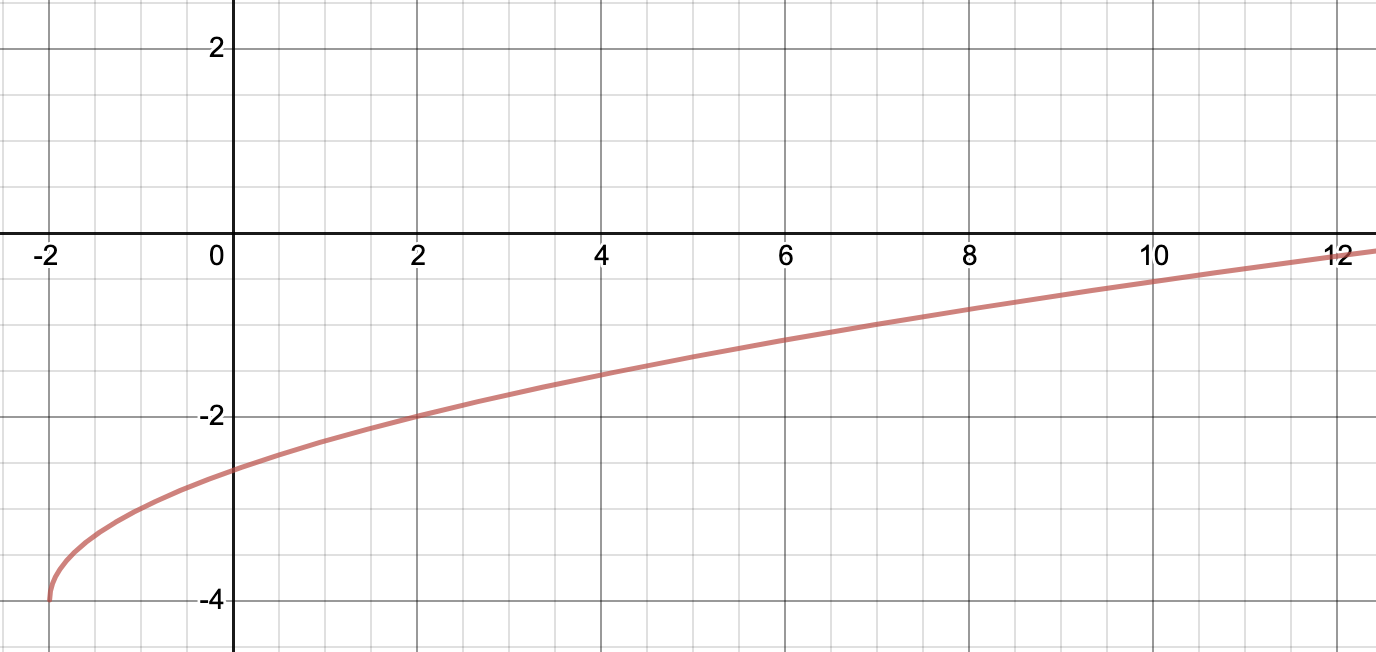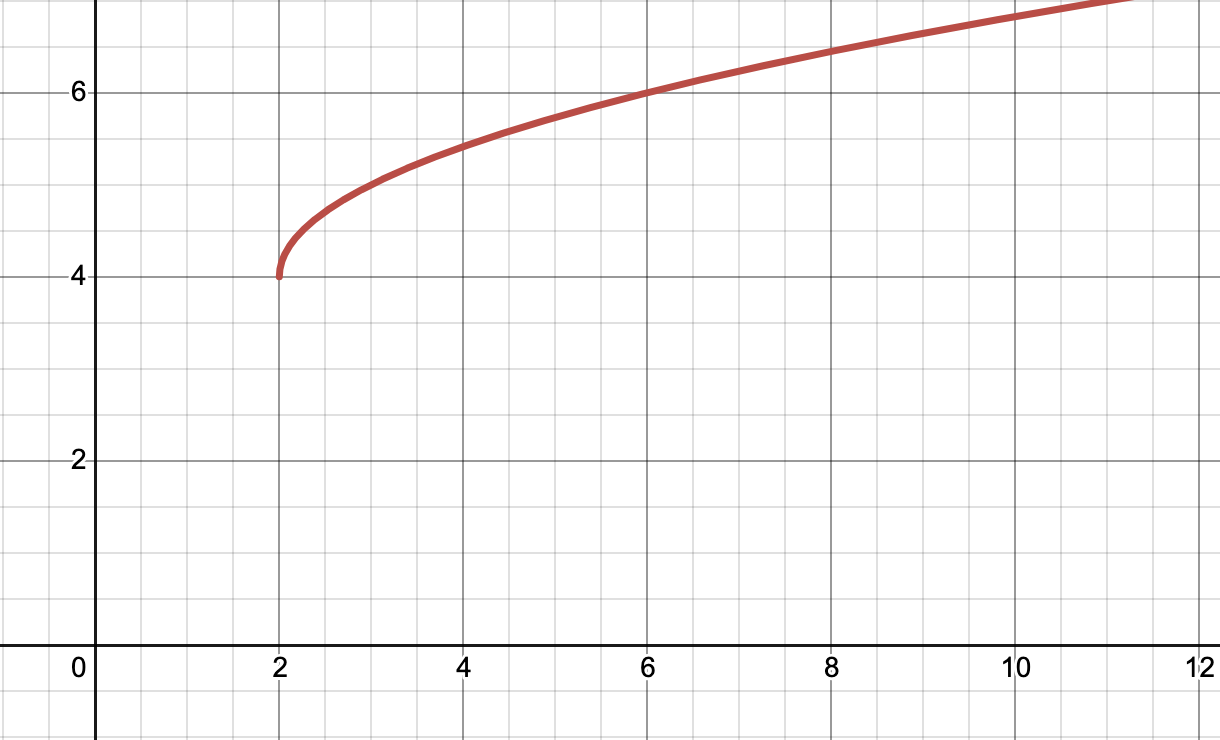Correct answer:Explanation:

The graph ofis:This graph is translated 2 units left and 4 units down from the graph of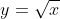, which looks like: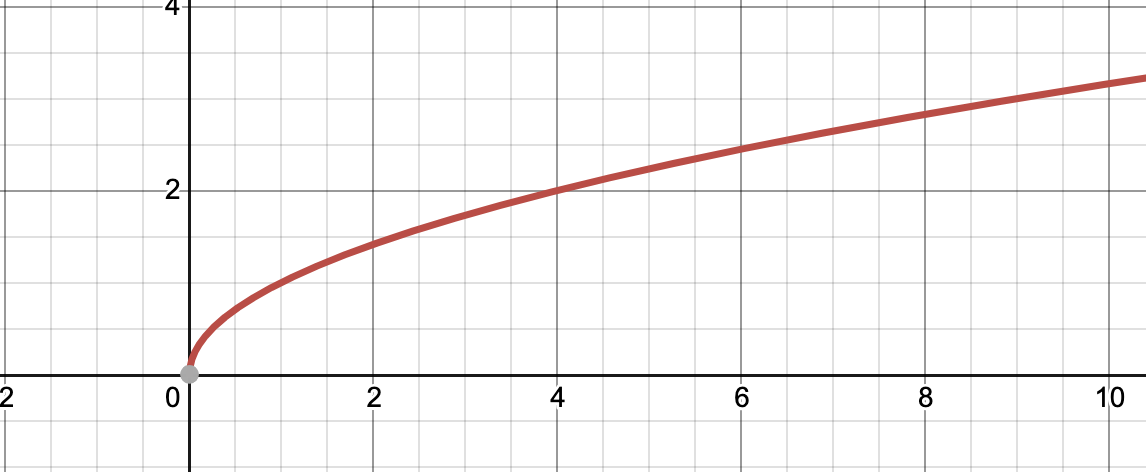The number 4 and its associated negative sign, which fall outside of the square root, tell us that we need to shift the graph down four units. If you take what's under the square root, set it equal to 0, then solve, you'll get x=-2, which you can interpret as being shifted left two units.

Each of the graphs below that are incorrect either improperly shift the graph right (instead of left), up (instead of down), or both right and up.

### Example Question #4 : Graph Square Root, Cube Root, And Piecewise Functions

Is the following graph a function?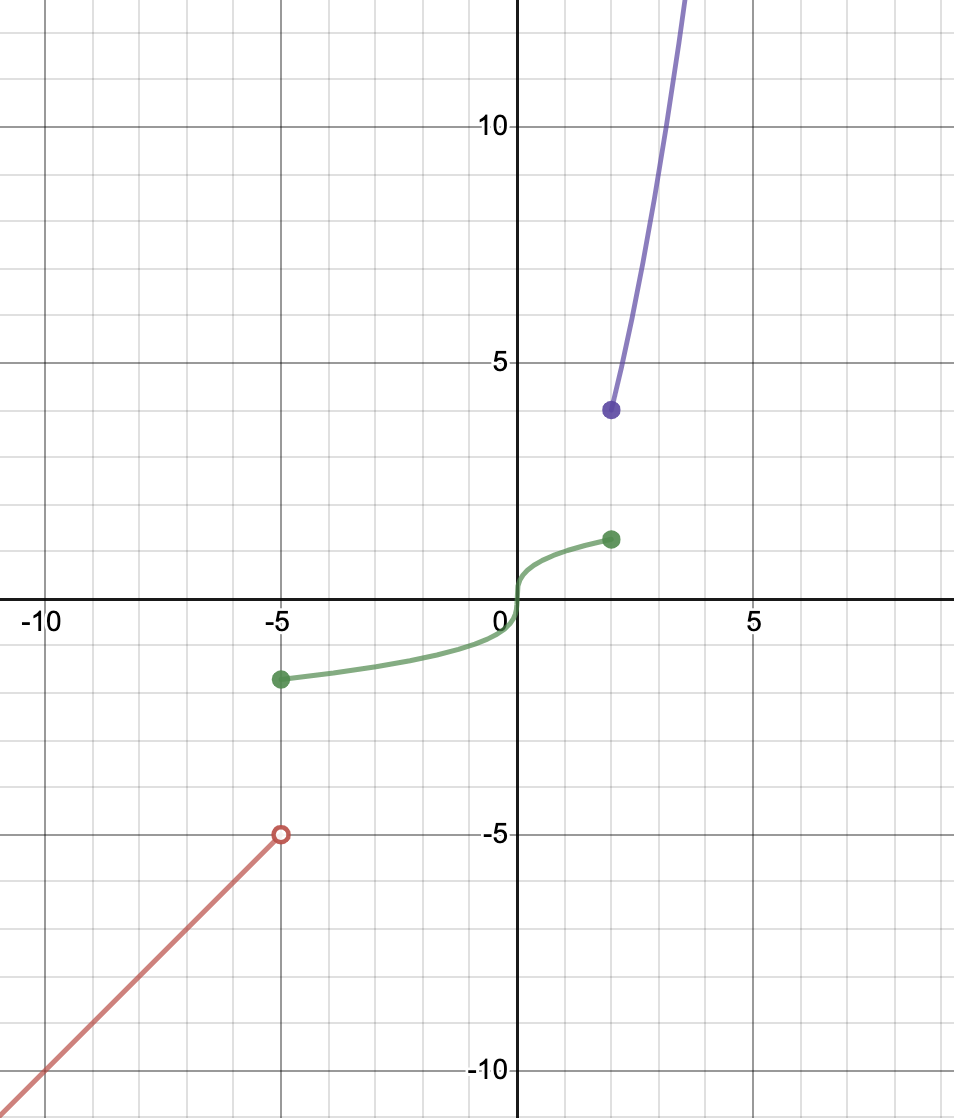Possible Answers:

No

Yes

Correct answer:

No

Explanation:

The above graph is not a function. In order to be a function, the graph must pass the vertical line test. If you look at x=2, you can see that x=2 is a value on both the green graph and the purple graph. Therefore it fails to pass the vertical line test and is not a function.

### Example Question #5 : Graph Square Root, Cube Root, And Piecewise Functions

Is the following graph a function?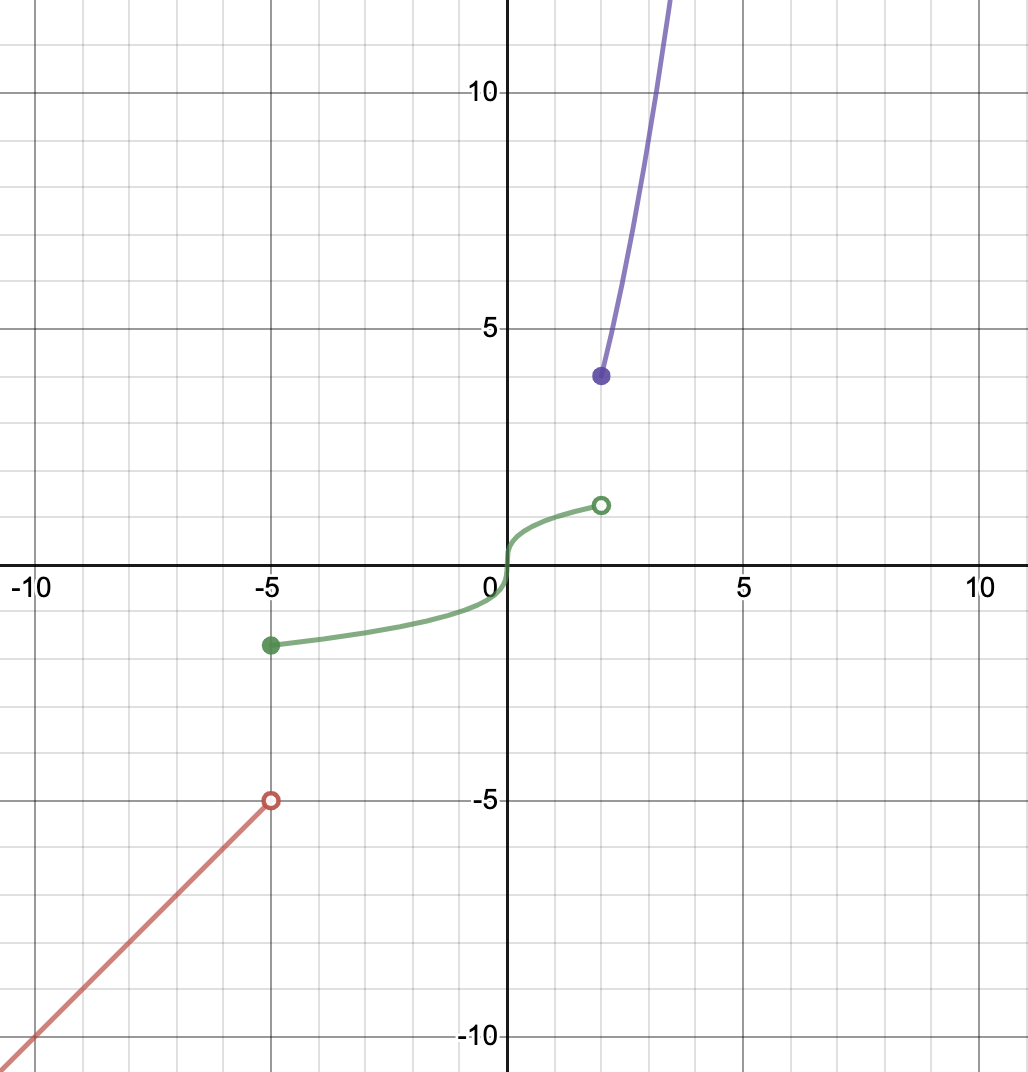Possible Answers:

Yes

No

Correct answer:

Yes

Explanation:

This graph is a function. We call this type of function a piecewise (or step) function. This type of function is defined in a certain way for certain x values, and then in a different way for different x values. However, it still passes the vertical line test, so it is a function!

### Example Question #6 : Graph Square Root, Cube Root, And Piecewise Functions

What is the domain and range of this piecewise function?Possible Answers:

Domain: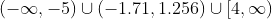Range: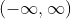Domain:Range:Domain: all real numbers

Range: all real numbers

This is not a function and therefore cannot have a domain and range.

Correct answer:

Domain:Range:Explanation:

Firstly, this is a function as it passes the vertical line test.

To find the domain, begin at the far left of the graph, starting with the red portion. You can trace along it continuously until you get to the point (-5,-5). At this point, it jumps upward to the green graph. While the y values jump, there is no gap in the x values. You can trace from left to right along the green graph until you get to x=2. Then, the graph jumps up to the purple values. Once again, there is no gap in the x values. The purple graph is continuous and goes onwards to positive infinity. Therefore, the domain of this graph is all real numbers or.

Next, let's start again at the bottom of the graph (in red), this time looking at the y values. We begin at negative infinity and can trace continuously until we get to the point (-5,-5). There is a discontinuity between the red and green graphs' y values. We use the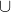symbol ("union") to connect the disconnected parts of the graph. The green graph picks up at -1.71 and the y values continue upward until they get to 1.256. Once again, there is a discontinuity in the range, so we again use the union symbol. The y values pick back up at the point (2,4) and continue upwards to infinity. Therefore the range of this function is.

### Example Question #7 : Graph Square Root, Cube Root, And Piecewise Functions

Which of the following is the graph of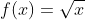?

Possible Answers: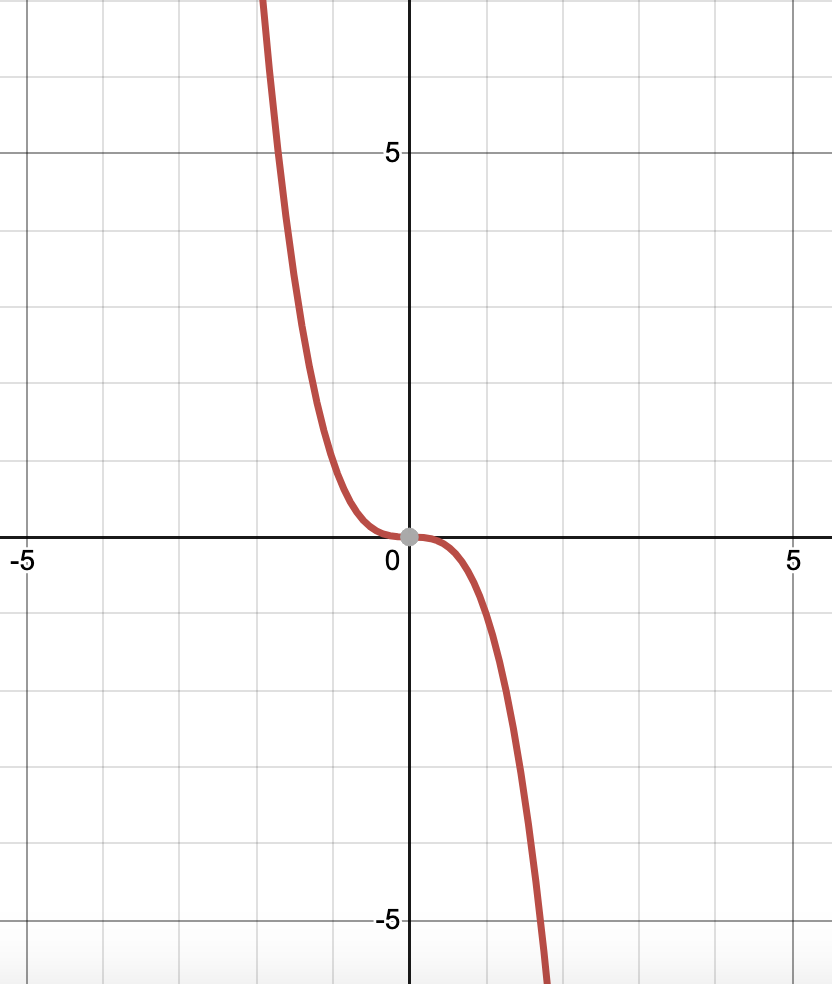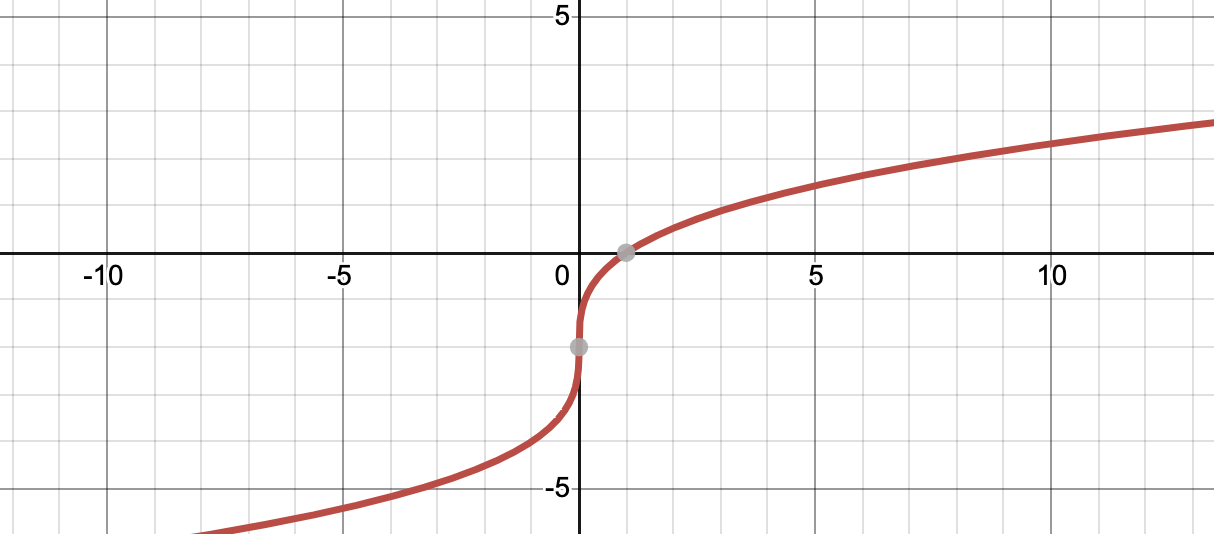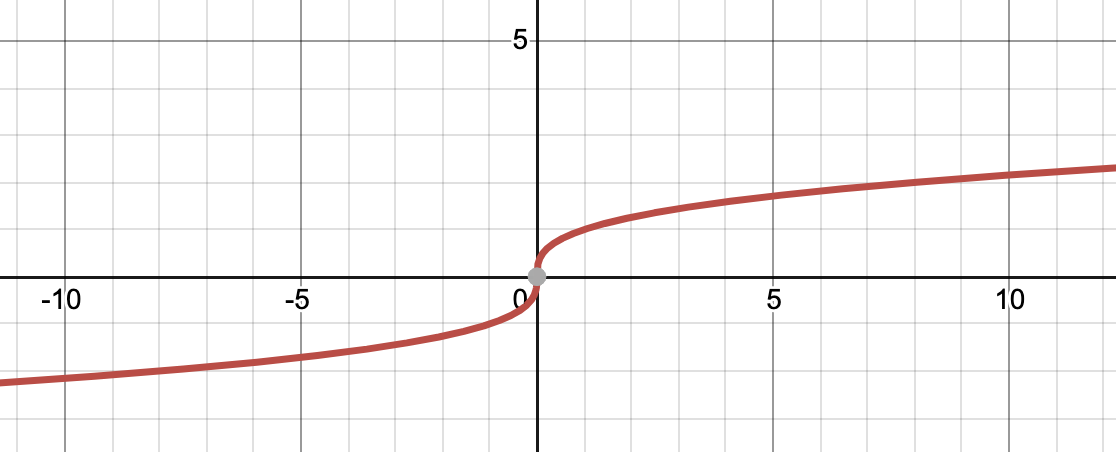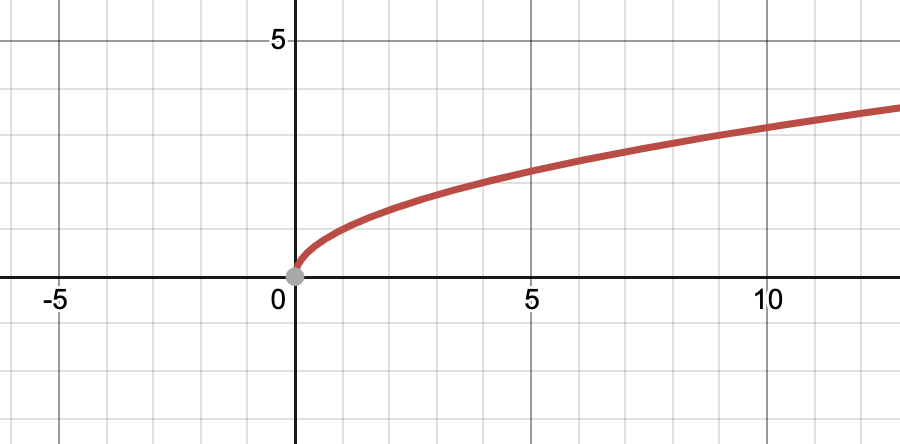Correct answer:Explanation:

The graph ofisKeep in mind that you cannot put a negative number under a square root and get a real number answer. If we tried to plug in -2 for x, it would be undefined. That is why the graph only shows positive values of x.

The other graphs pictured below are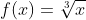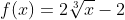, and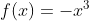.

### Example Question #8 : Graph Square Root, Cube Root, And Piecewise Functions

Which of the following is the graph of?

Possible Answers:Correct answer:Explanation:

The graph ofis.

The other graphs shown are those of, and.

### Example Question #9 : Graph Square Root, Cube Root, And Piecewise Functions

Which of the following is the graph of?

Possible Answers:Correct answer:Explanation:

The graph ofisThe other graphs pictured are, and.

### Example Question #10 : Graph Square Root, Cube Root, And Piecewise Functions

State the x-intercept, y-intercept, domain, and range of the function, pictured below.Possible Answers:

x-intercept: none

y-intercept: none

Domain: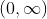Range:x-intercept: none

y-intercept: none

Domain: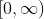Range:x-intercept: x=0

y-intercept: y=0

Domain:Range:x-intercept: x=0

y-intercept: y=0

Domain:Range:Correct answer:

x-intercept: x=0

y-intercept: y=0

Domain:Range:Explanation:

This graph intersects the x axis only once and the y axis only once, and these happen to coincide at the point (0,0). Therefore the x-intercept is at x=0 and the y-intercept is at y=0. The graph will go on infinitely to the right. However, the leftmost point is (0,0.) Likewise, the graph will go infinitely high, but its lowest point is also (0,0). Therefore, its domain isand its range is also.

← Previous 1

### All Algebra II Resources